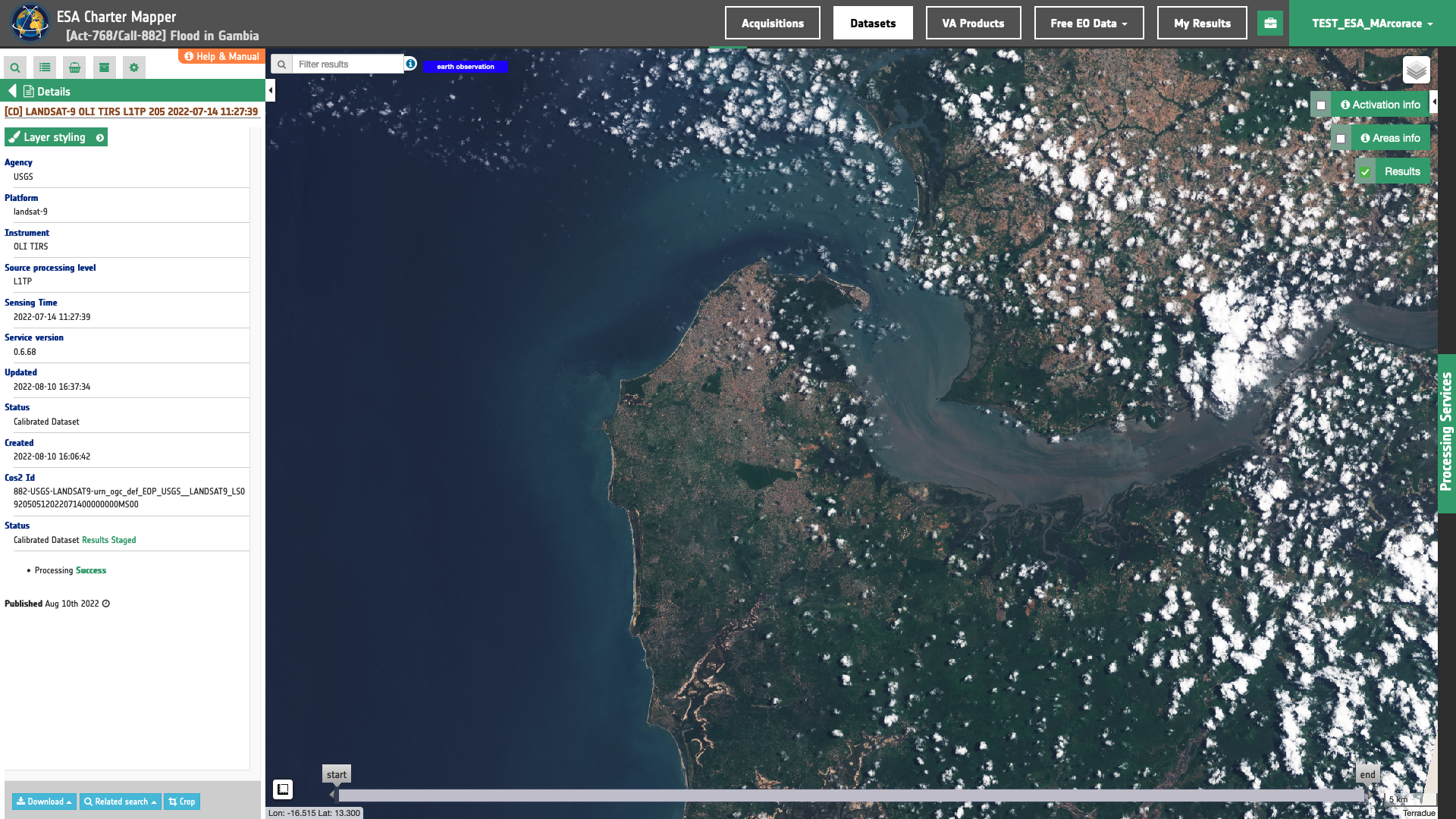# Landsat-9

Landsat-9 panchromatic and multispectral EO data products are calibrated using the Optical Products Calibration (OPT-Calib) service. The process applied is the same for PAN and MS acquisitions.## Input

The input is an ingested Landsat-9, find details here.

## DN to Reflectance

Reflective band DN’s are converted to TOA reflectance using the rescaling coefficients in the MTL file1:

$R(b)′= M_b \times DN + A_b$

where:

$$R(b)'$$ is the Top of Atmosphere planetary reflectance, without correction for solar angle.

Note that $$R(b)'$$ does not contain a correction for the sun angle.

• $$M_b$$ is the band-specific multiplicative rescaling factor from the metadata (REFLECTANCE_MULT_BAND_x, where x is the band number)

• $$A_b$$ is the band-specific additive rescaling factor from the metadata (REFLECTANCE_ADD_BAND_x, where x is the band number)

Top of Atmosphere reflectance with a correction for the sun angle is then:

$R(b) = R(b)' \times cos(θ_{SZ}) = R(b)' \times sin(θ_{SE})$

where:

• $$R(b)$$ is the TOA planetary reflectance

• $$θ_{SE}$$ is the local sun elevation angle. The scene center sun elevation angle in degrees is provided in the metadata (SUN_ELEVATION).

• $$θ_{SZ}$$ is the local solar zenith angle; $$θ_{SZ} = 90° - θ_{SE}$$

## Output

The output is a STAC item GeoJSON2 having a similar structure to the Landsat-8 one available here.

1. USGS, Using the USGS Landsat Level-1 Data Product, available at: https://www.usgs.gov

2. Radiant Earth Foundation, STAC item specifications, a single spatiotemporal asset as a GeoJSON feature plus datetime and links, available at:https://github.com